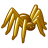# VB error in model

356
6
Jump to solution
01-10-2013 05:59 AMOccasional Contributor
We have recently upgraded from Desktop 9.3 to 10.0, and now are receiving an error when running a model. Unknown if the error is due to the upgrade or not. I do know that we had problems using the field calculator upon upgrading, if a custom toolbox was saved with the 9.3 field calculator. Here is the modelbuilder error:
`General error executing calculator. ERROR 999999: Error executing function. Expected end of statement Failed to execute (Calculate Field).`
When running the 'Calculate Field' geoprocessor with the VB code below.
`Dim dblISOLEVEL As Double Dim lngJoinCnt As Long Dim dblZLEVEL as Double  lngJoinCnt = [Join_Count] dblZLEVEL = [ZLEVEL]  if (lngJoinCnt = 1) and (dblZLEVEL = 0) then dblISOLEVEL = -1 elseif (lngJoinCnt > 1) and (dblZLEVEL = 0) then dblISOLEVEL = dblZLEVEL elseif (lngJoinCnt = 1) and (dblZLEVEL = 5) then dblISOLEVEL = 0 elseif (lngJoinCnt > 1) and (dblZLEVEL = 5) then dblISOLEVEL = dblZLEVEL else  dblISOLEVEL = dblZLEVEL end if`
Tags (3)
1 Solution

Accepted SolutionsOccasional Contributor
Yes, had to even go a bit further:
`Dim dblISOLEVEL Dim lngJoinCnt Dim dblZLEVEL  lngJoinCnt = CLng([Join_Count]) dblZLEVEL = CDbl([ZLEVEL])`
6 RepliesFrequent Contributor
So this is VBScript code block within the field calculated in a model?
I think you variable declaration is wrong - only variant types are instantiated according to the note in the web help, see below:

"VBScript does not allow you to explicitly declare any data types; all variables are implicitly Variant. Statements like Dim x as String should be removed or simplified to Dim x."

Calculate Field examples
Resource Center » Professional Library » Data Management » Geographic data types » Tables » Calculating field values
http://help.arcgis.com/en/arcgisdesktop/10.0/help/index.html#//005s0000002m000000

So test changing your var declare to the below, see if that works (leave the testing to you)---
Good luck!

-Wayne

`Dim dblISOLEVELDim lngJoinCntDim dblZLEVEL`Occasional Contributor
Gotcha. Thanks.Frequent Contributor
So did that solve your problem?
If so, please mark this as 'answered'.

Thanks,
WayneOccasional Contributor
Yes, had to even go a bit further:
`Dim dblISOLEVEL Dim lngJoinCnt Dim dblZLEVEL  lngJoinCnt = CLng([Join_Count]) dblZLEVEL = CDbl([ZLEVEL])`Frequent Contributor
Yes well if the 'variant' data type can hold all those types, I suppose you'd be fine if you assigned the values directly already in the proper format, i.e. no need to use the conversion functions (CLng and CDbl) if you pass the values in that way.

So a val from an integer field could be passed directly without CLng; a val from a double field could be passed in... etc.

Hope you got it working okay.Occasional Contributor
Yes Sir, Mr. Whitley. It is working now.  Thanks!